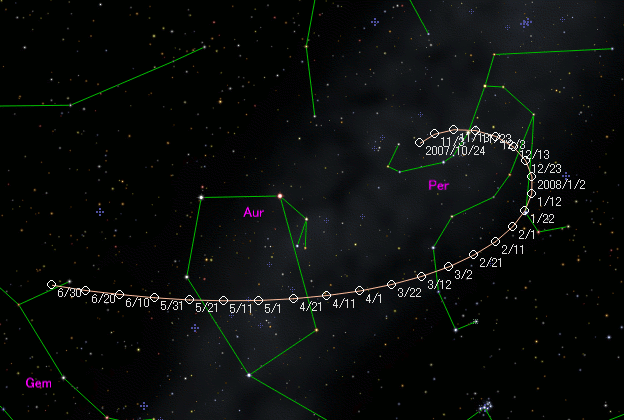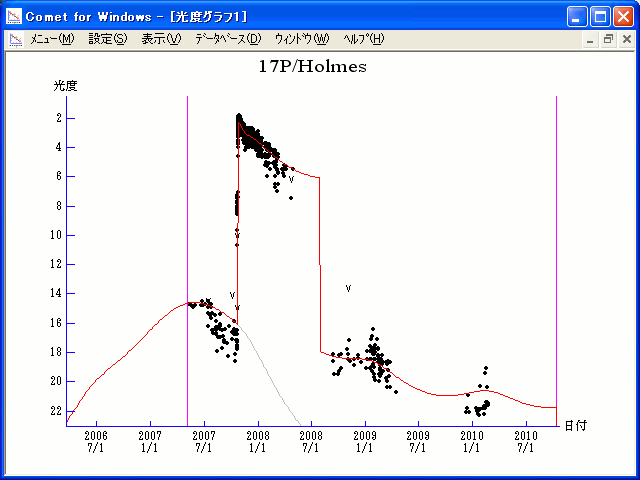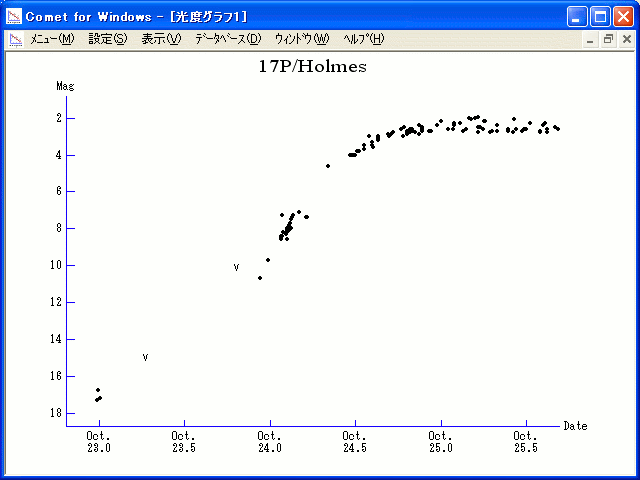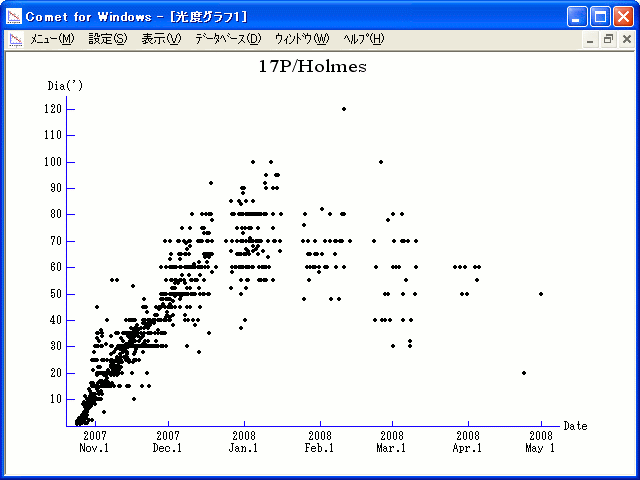# 17P/Holmes (2007)###Pictures###Orbital Elements

```The following orbital elements are taken from MPC 51822:

Epoch 2007 May 20.0 TT = JDT 2454240.5
T 2007 May 4.4995 TT                                    MPC
q   2.053169             (2000.0)            P               Q
n   0.1432508      Peri.   24.2585      +0.9756506      +0.1267918
a   3.617468       Node   326.8675      -0.2124462      +0.7491399
e   0.432429       Incl.   19.1132      +0.0545208      +0.6501640
P   6.88
From 139 observations 1964-2001, mean residual 0".7.  Nongravitational
parameters A1 = +0.19, A2 = +0.0287.
```

###Finding Charts###Magnitudes Graph

```        m1 =   1.5 + 5 log d + 35   log r  [     ,172.5]  (             - 2007 Oct. 24)
m1 = -18.2 + 5 log d + 50   log r  [172.5,198  ]  (2007 Oct. 24 - 2007 Nov. 18)
m1 =   0.0 + 5 log d +  5.0 log r  [198  ,450  ]  (2007 Nov. 18 - 2008 July 27)
m1 =   3.5 + 5 log d + 20   log r  [450  ,     ]  (2008 July 27 -             )

* Gray curve is:  m1 = 1.5 + 5 log d + 35 log r
```##### The orbital elements are published at the MPC Ephemerides and Orbital Elements. The charts are made with StellaNavigator Ver.8 (AstroArts). The magnitudes graphs are made with Comet for Windows.